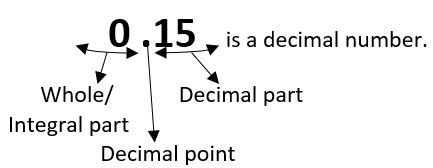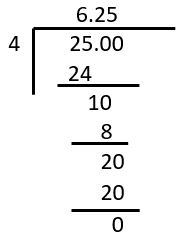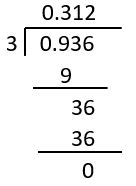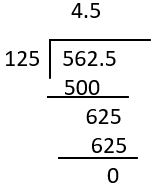## LetsPlayMaths.Com

WELCOME TO THE WORLD OF MATHEMATICS

# Class 7 Decimals

Decimal Fractions

Convert Decimal Number into Fraction

Convert Fraction into Decimal Number

Types of Decimals

Comparison of Decimals

Subtraction of Decimals

Multiplication of Decimals

Division of Decimals

Decimals Test

Decimals Worksheet

## Decimal Fractions

Decimal number system consists a fraction whose denominator can be either ten, hundred, thousand or a power of ten can also be represented as decimal numbers. A decimal number system has two parts which are separated by a point/dot(.) known as decimal point. The number to the left of decimal point is known as Whole part or Integral part. The number to the right of decimal point is known as Decimal part.The number of digits contained in the decimal part are known as the number of Decimal places.## Reading Decimal Numbers

• The decimal numbers are read from left to right. The left part i.e. the integral part presented by it's value where as the decimal part are read by naming each digit individually.
• The decimal point will be read as "point"
• If there is no number before the decimal point then it will be read as zero

Values ... Thousands Hundreds Tens Ones Tenths(110) Hundredths(1100) Thousandths(11000) ...
25.4 2 5 4
256.35 2 5 6 3 5
5782.056 5 7 8 2 0 5 6
25.004 2 5 0 0 4

Example 1. 4235.678 = 4000 + 200 + 30 + 5 + 610 + 7100 + 81000

= 4000 + 200 + 30 + 5 + 0.6 + 0.07 + 0.008

It will be read as : four thousand two hundred thirty five point six seven eight.

Example 2. 0.06 = zero point zero six / point zero six

## Convert Decimal Number into Fraction

• Write the provided decimal without the decimal point in the numerator of the fraction.
• In the denominator part of the fraction, write 1 followed by as many number of zeros as there are decimal places in the given decimal.
• At last reduce the fraction to it's simplest form.

Example 1. Convert 4.64 into fraction.

Solution. 4.64 = 464100 = 23250 = 43250

Example 2. Convert 0.354 into fraction.

Solution. 0.354 = 3541000 = 3541000 = 177500

## Convert Fraction into Decimal Number

Method 1

• If a denominator is given 10/100/1000/any power of 10 in a given fraction, then write the numerator part separately as decimal part.
• Mark the decimal point in the number of decimal part followed by number of zeroes in the denominator starting from the unit digit by counting from extreme right to left.
• The numbers of digit after decimal must be equal with the number of zeroes in denominator.

Example 1. Convert 36510 to decimal form.

Solution. 36510 = 36.5
As there is one zero in denominator so the decimal point is in between 36 and 5.

Example 2. Convert 1481000 to decimal form.

Solution. 1481000 = 0.148
As there is three zeros in denominator so the decimal point is placed before 148.

Example 3. Convert 469100000 to decimal form.

Solution. 469100000 = 0.00469

Method 2

• When the denominator of the given fraction is other than 10,100, any power of 10 then simply divide the numerator with denominator.
• Mark a decimal point in the quotient and dividend just after the division of unit digit is completed.
• Divide again and put any numbers of zeroes (one by one) in the dividend as well as remainder in order to complete the division.
Let's see some exmaples.

Example 1. Divide 25 by 4.

Solution. 25 ÷ 4254 = 6.25

## Types of Decimals

Decimals can be classified into different kinds.

1. Like Decimals
2. Unlike Decimals
3. Terminating Decimals
4. Non-terminating/Non-repeating Decimals
5. Recurring Decimals

Like Decimals: The Decimals consisting the same number of decimal places are called like decimals.

Example. 8.34, 20.78, 6.22 are like decimals as each of having two decimal places.

Unlike Decimals: The decimals consisting different number of decimal places are called Unlike Decimals.

Example. 7.353, 55.32, 65.4 are unlike decimals as each of having diff. decimal places.

Terminating Decimals: When a fraction is completely divisible and there is a finite number of digits after the decimal points in quotient are called as Terminating Decimals.

Example. 31.764 = 7.94 is a terminating decimal.

Non-terminating/Non-repeating Decimals: When a fraction is not completely divisible and there is not having an end term after the decimal points are called as Non-terminating Decimals.

Example. 10013 = 7.69230...

Recurring Decimals: When a fraction is not completely divisible and the decimal after digits are periodic or repeat again and again are known as Recurring or Repeating decimals.

Example. 3522 = 1.59090909...

## Comparison of Decimals

First convert the decimals into like decimals. Then compare the digits of whole part. If the whole part is same then compare digits of decimal part in correspondence to their tenth, hundred, thousand etc. value.

Example 1. Compare 7.84 and 7.9.

Solution. Let's convert both the decimals into like decimals.

Now both the like decimals are 7.84 and 7.90.

Here, whole part of both the decimals are same i.e. 7. So, we have to compare the decimal part.

90 > 84

Hence, 7.9 > 7.84

Example 2. Compare 7.84, 98.4, 6.403.

Solution. Convert all the three decimals into like decimals.

7.840, 98.400, 6.403

Now compare the whole part of the three decimals.

98 > 7 > 6

Hence, 98.4 > 7.84 > 6.403.

## Addition of Decimals

• Convert the decimals into like decimals
• Write them in the tabular form and add them as normal addition

Example 1. Add 4.80, 12.043, 246.94

Solution. 4.80 + 12.043 + 246.94

Convert all the three decimals into like decimals.

= 4.800 + 12.043 + 246.9404.80 + 12.043 + 246.94 = 263.783.

## Subtraction of Decimals

• Convert the decimals into like decimals
• Arrange them vertically in the tabular form and subtract them as normal subtraction

Example 1. Subtract 0.474 from 5.

Solution. 5 − 0.474

Convert both the decimals into like decimals.

= 5.000 − 0.4745 − 0.474 = 4.526

## Multiplication of Decimals

• Multiplication by 10,100,1000 or any power of 10
• Multiplication of decimals into another decimals

Multiplication by 10,100,1000 or any power of 10

We have to shift the decimal point to the right by as many digits as there are zeroes in 1. Let's see some examples.

Example 1. Multiply 521.12 by 10.

Solution. 521.12 x 10 = 5211.2

Example 2. Multiply 521.12 by 100.

Solution. 521.12 x 100 = 52112.0 = 52112

Multiplication of decimals into another decimals

• Multiply the decimals in a normal way by ignoring the decimals.
• Count the numbers of digit after decimal point of each number and add them.
• Start from the right of the product and count number of places equal to the total number of places got in previous step and place the decimal point.

Example 1. Multiply 12.3 and 3.4.

Solution. 12.3 x 3.4

Multiply both the decimal by ignoring their decimal point.

123 x 34 = 4182

Coun the number of digits present after the decimal point of both the decimal number.

= 1 + 1 = 2

Starting from the right of the product 4182 and count up to 2 places, then place the decimal point.

Hence the answer is 41.82.

## Division of Decimals

• Division by 10,100 or any power of 10
• Decimal division by a whole number
• Division of Decimal by another Decimal

Division by 10,100 or any power of 10

Shift the decimal point to the left by as many digits as there are number of zeroes in divisor.

Example 1. Divide 756.78 by 10.

Solution. 756.78 ÷ 10

By shifting decimal point one digit to the left we will have the answer

756.78 ÷ 10 = 75.678

Example 2. Divide 756.78 by 100.

Solution. 756.78 ÷ 100

By shifting decimal point two digits to the left we will have the answer

756.78 ÷ 100 = 7.5678

Decimal division by a whole number

Divide the decimal number by a whole number by simply ignoring decimal points. Mark the decimal point in quotient by completing the division in the dividend. Let's see some examples.

Example 1. Divide 0.936 by 3.

Solution. 0.936 ÷ 3Division of Decimal by another Decimal

Convert the divisor decimal number into a natural number by shifting the decimal point by the same number of places in the right direction in the divisor and dividend both. Divide this new dividend by the new divisor in the usual way of division.

Example 1. Divide 562.5 by 12.5.

Solution. 562.5 ÷ 12.5## Class-7 Decimals Test

Decimals Test - 1

Decimals Test - 2

## Class-7 Decimals Worksheet

Decimals Worksheet - 1

Decimals Worksheet - 2

Decimals Worksheet - 3

Decimals Worksheet - 4